# Tutorial: How To Obtain Relative Accurate Results From Stress Analysis in Autodesk Inventor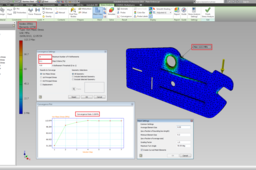FEA is not a simple mathematical operation.

Instead it is a computer-based iterative numerical technique along with inevitable discretization errors, as a function of elements.

Therefore these errors can be reduced by...
specifying more elements (H-refinement)
or
employing a higher order of polynomial at each iteration (P-refinement).

Effectively more computational power is required to solve complex shaped geometries with high dense mesh (Elements).

But how dense should be my mesh to get appropriate results without wasting hours on simulation ???

This is the Golden Question!

... There is a criterion for that, called Converged solution...

In plain words the mesh does not need any further refinement (denser mesh) as long as the solution is "Converged".

The solution is assumed to be "Converged" when the results of the polynomial eq. does not change according to a prespecified tolerance e.g. 10% Good Results, 0.1% Great Results.

So, let me share some information how to manipulate the mesh for a model along with three examples.

As a conclusion we will enhance your confidence regarding the results you get, whether are relatively accurate or not (Low Percentage of Discretization Errors) without wasting hours on simulation!!!

Don't Forget the simulation results are nothing without any experimental results to compare with.

1. ### Step 1:

Assuming that you applied the constraints and the loading according to your problem and you are 1 step before hit the Run button of the simulation!!!

Namely there are two ways to follow for a converged solution

The Manual refinement & The Automatic refinement.

We will first explain the features of each dialogue depicted below and then we will see how they affect our solution.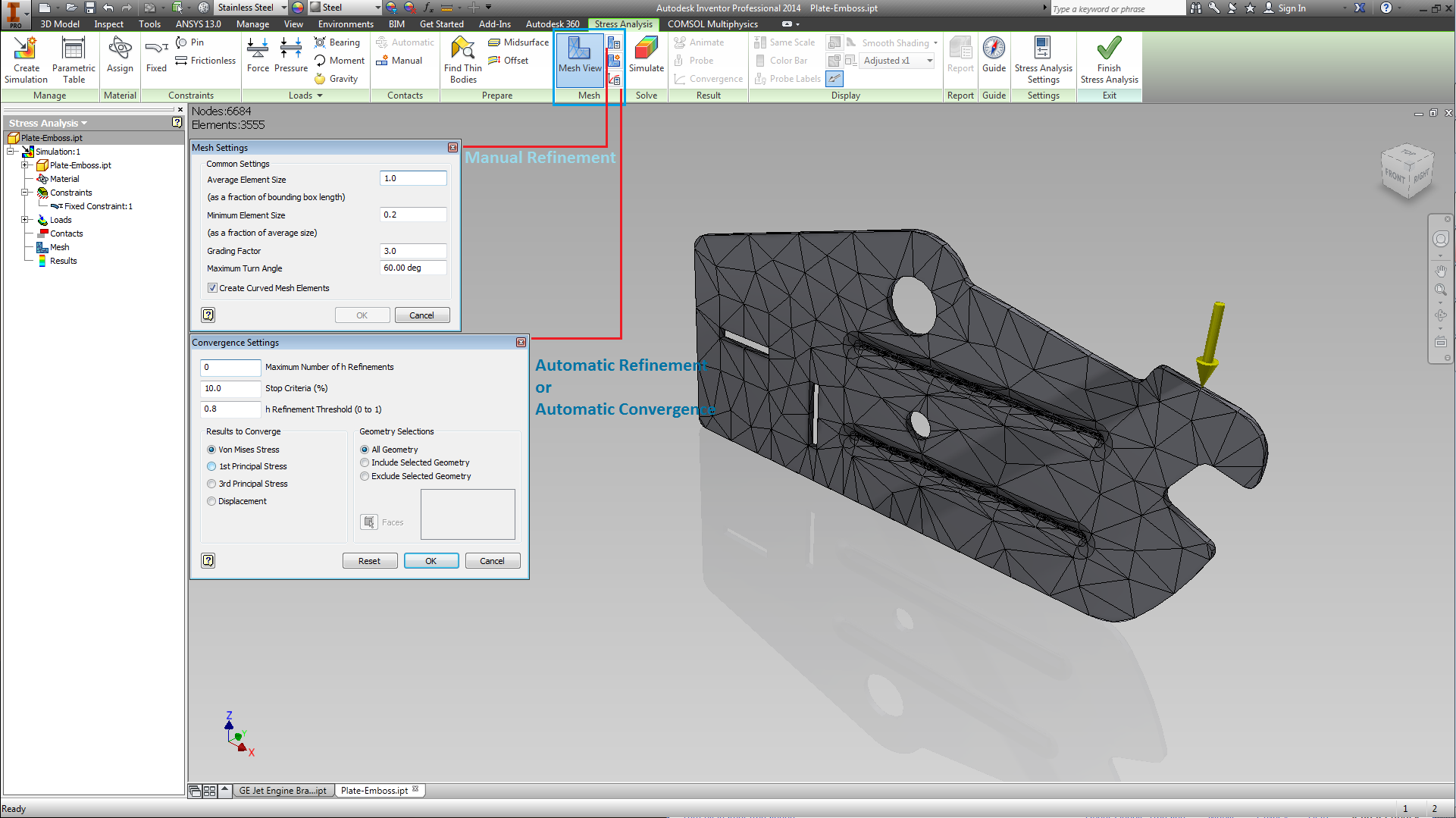2. ### Step 2:

Manual refinement...

The Average Element Size.
This Parameter cannot have a value greater than >1.
An Example can give you an idea of how you can calculate the length of it.
Size of mesh element = Longest parameter (dimension) of object * Average Element Size.
Depends on how simple or complex but most important how BIG is your model I would suggest an Initial value of of 0.4.
This is a general rule that will keep your simulation to run for a short time while you will capture some of the most critical areas of interest.

Minimum Element Size.
This parameter ensures that NO element below that length will appear in our model
Is recommended to be around 0.2

Produce a more uniform mesh around curves that is always desirable for the smooth operation of the numerical technique.
It is recommended to be around 1.5 depends on the transition of the mesh

Maximum Turn Angle.
It is true that a smaller value will conclude to a denser mesh.
It is recommended for this parameter to be between 30 & 60 deg.

Curved Mesh Element.
Ensures that curved elements will be used to represent a more accurate solution than straight elements around circular features.

So far you saw how you can manipulate the mesh of your model... as depicted below!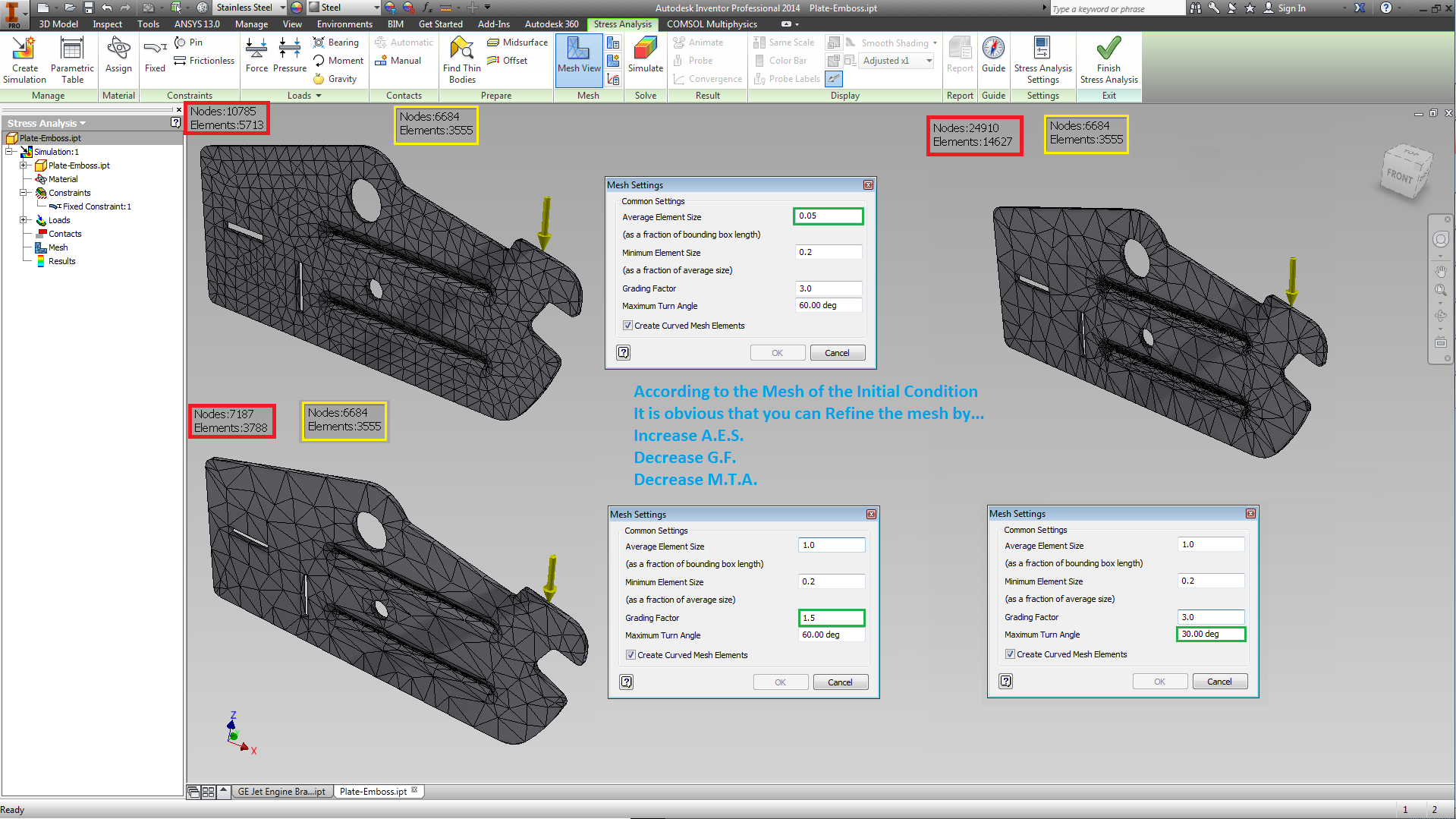3. ### Step 3:

Automatic refinement...

Maximum Number of h Refinements
This parameter specifies the number of element refinements based around maximum stresses.
Keep that below 5 as may result in stress singularities.

Stop Criteria (%)
This is the tolerance that you allow between the two solutions in order to assumed converged.
(Remember the we speak about an iterative numerical model)
It is obvious that a lower % will need more iteration and consequently more time.
As mentioned before... 10% Good Results, 2% Great Results (For Inventor).

h Refinement Threshold (0 to 1)
This value is set to 0.75 that means 25% of elements around peak stress regions are likely to be refined
Therefore, smaller value leads more elements around peak stress regions to get refined.

Results to Converge
In this section you can specify which results do you want to converge... Apparently is related to the Stop Criteria (%)
Tricky choice so let's stick with the Von Mises for now ;P

Geometry Selections
Use this section mainly to avoid stress singularities
The stress singularities are infinite stresses due to very small area almost=0
Generally is very important & you should be able to recognize these - Google will help ;P

The default Convergence Settings dialogue is depicted below the "Maximum Number of h Refinement" is set to 0.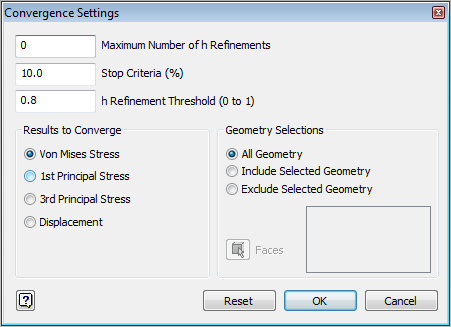4. ### Step 4:

Let's see now what exactly is happening on the background when you run the analysis along with the converged results and the Stop Criteria %

The H-P Convergence Process on the left as depicted below is the algorithm that solves your analysis. For the first three iterations the algorithm is trying to reduce the errors by employing a higher polynomial order.(there is no specified tolerance so you have to check you convergence rate every time for desirable results)
Additionally for these three iterations no refinement take place therefore any potential refinement for more accurate results has to be done manually by change parameters from the "Mesh Settings" (manual refinement of model).

As it can be seen from the Convergence plot, by setting the "Maximum Number of h Refinement" to 4 (Keep it below 5 is suggested),another 4 solution steps occur. If you assign all these solution steps to the algorithm on the left you can see how many refinements took place automatically.

It is obvious that your solution now is fluctuating in a very small interval (0.044%). Therefore you can be confident that the Mathematical model gave you the best result that could compute.

While on the manual refinement you need to do the following
1.Run analysis with Average Element Size of 0.1
2.Rerun analysis with Average Element Size of 0.05
3.Rerun analysis with Average Element Size 0.025
If the difference between the first and last analyses is within 10%, you can assume that your
results have converged.
(This last one is Copy - Paste from the reference book)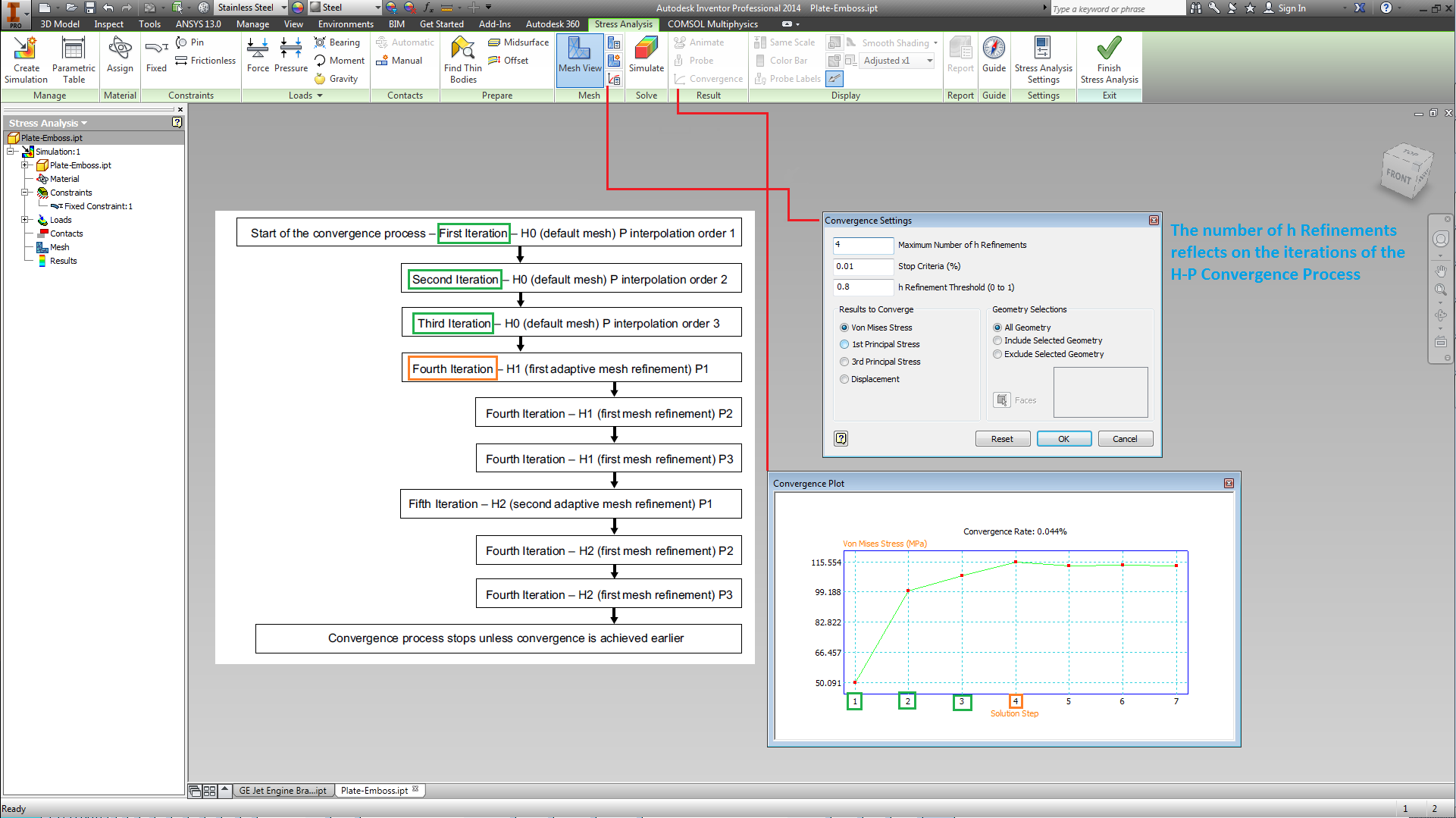5. ### Step 5:

Example 1

I run the analysis with the initial condition of the mesh settings (Too Rough Mesh) & no refinement.
I have got a ~8.5% Convergence rate that is not so bad.
However a refinement is always a good way to double check and reduce your Convergence rate even more.
What you need to check is...
The number of the Elements, 3570
Max VM = 110.5 MPa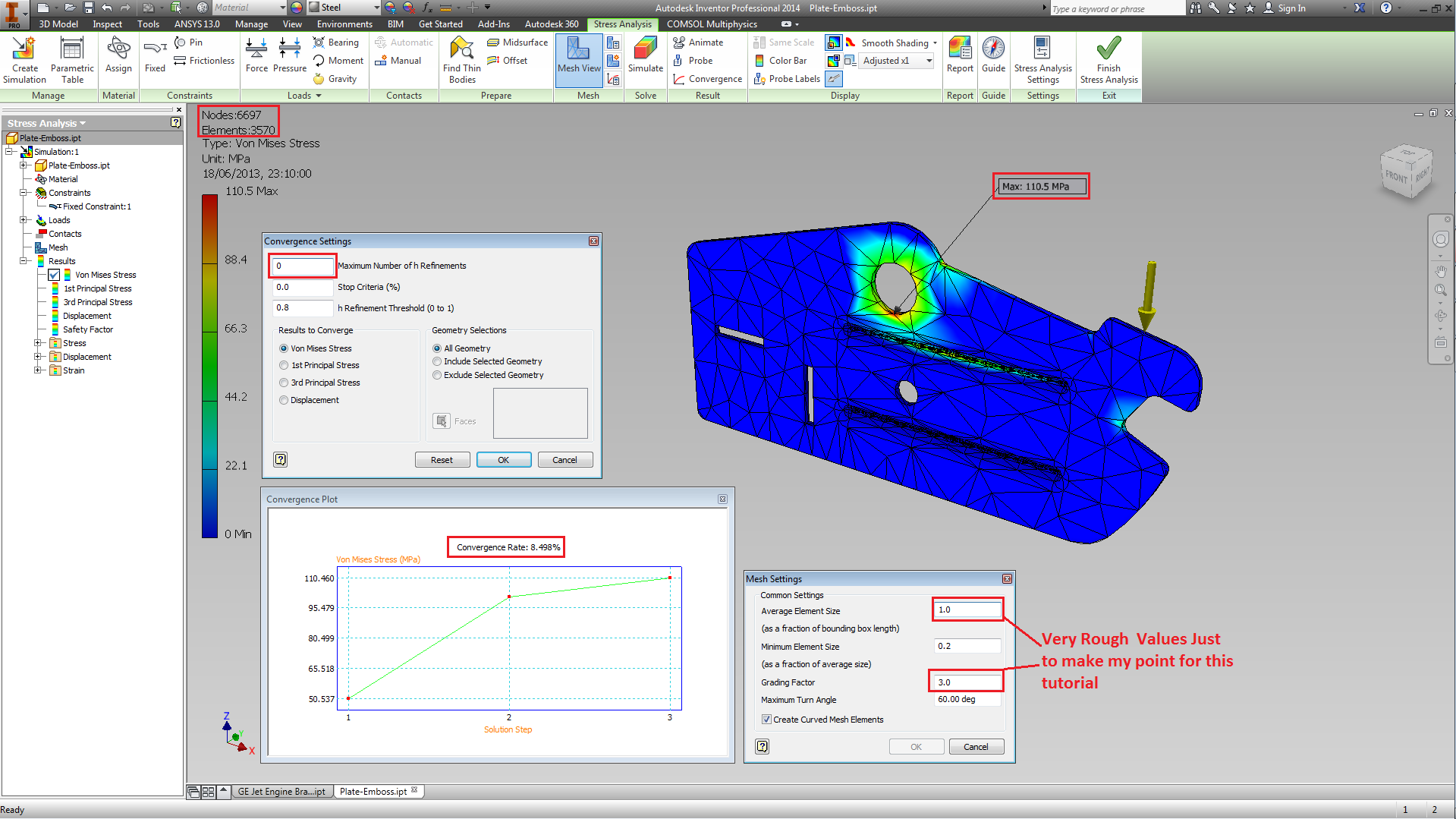6. ### Step 6:

Example 2

I rerun the analysis with a couple of changes in the mesh settings to get a more uniform not very dense mesh that will allow my convergence rate to drop even more...
What you need to check is...
I have got a ~8.064% Not big change.
The increment of the Elements, 5692
The Av. Element Size of 0.05 refinement,
And the max stress is now on a different point. Oops!
This is because of the Grading factor reduced (1.5) and a better calculation around the circumference obtained.
Max VM = 110.5 MPa
Previous point with Max VM has now ~ 88 MPa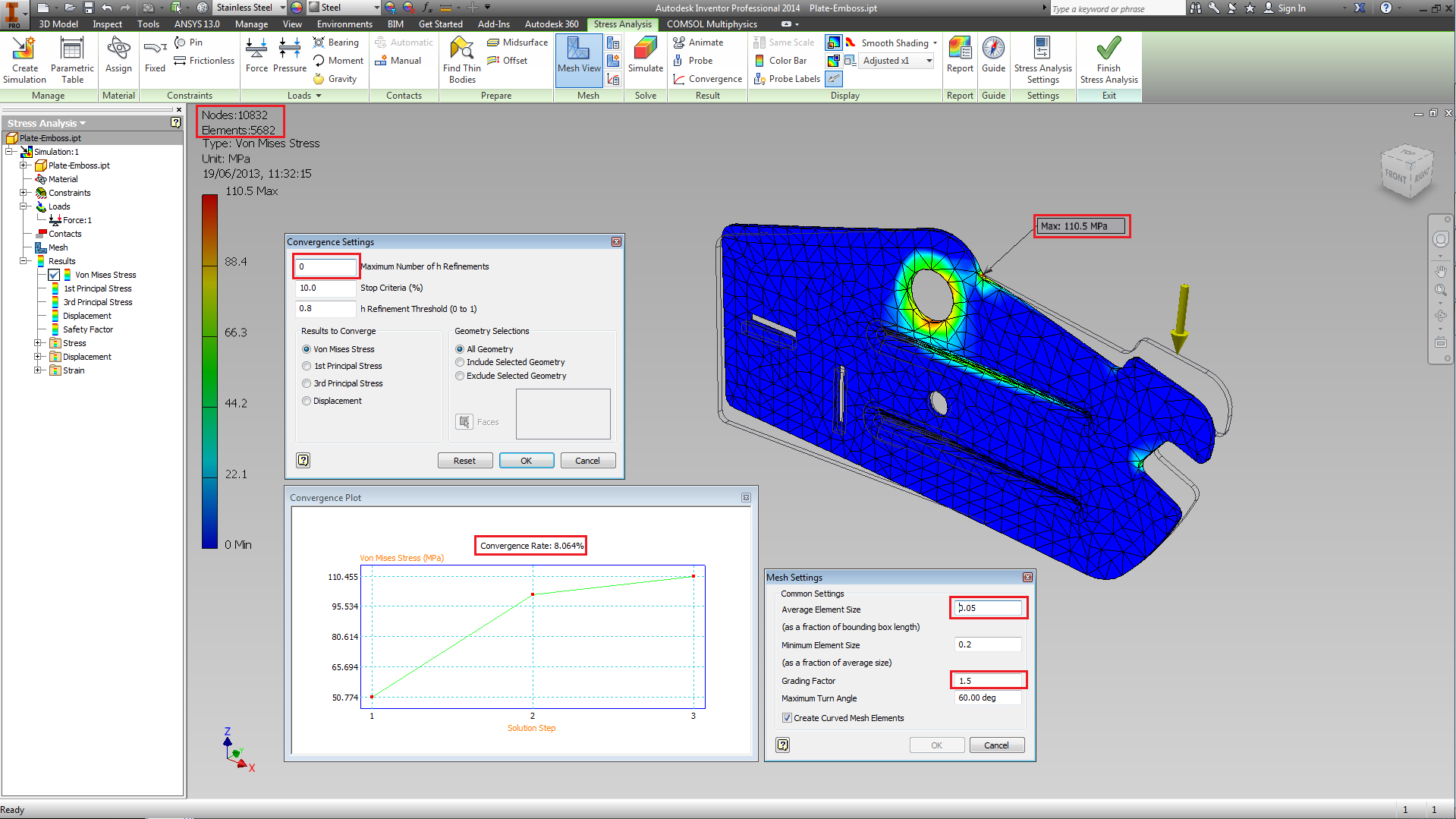7. ### Step 7:

Example 3

I rerun the analysis for third time while I am trying to bring my Convergence rate lower along with a nice mesh. I start the automatic refinements by setting this to 2.
What you need to check is...
The increment of the Elements, 8680
Max Turn Angle is 50 deg
Max VM = 113.2 MPa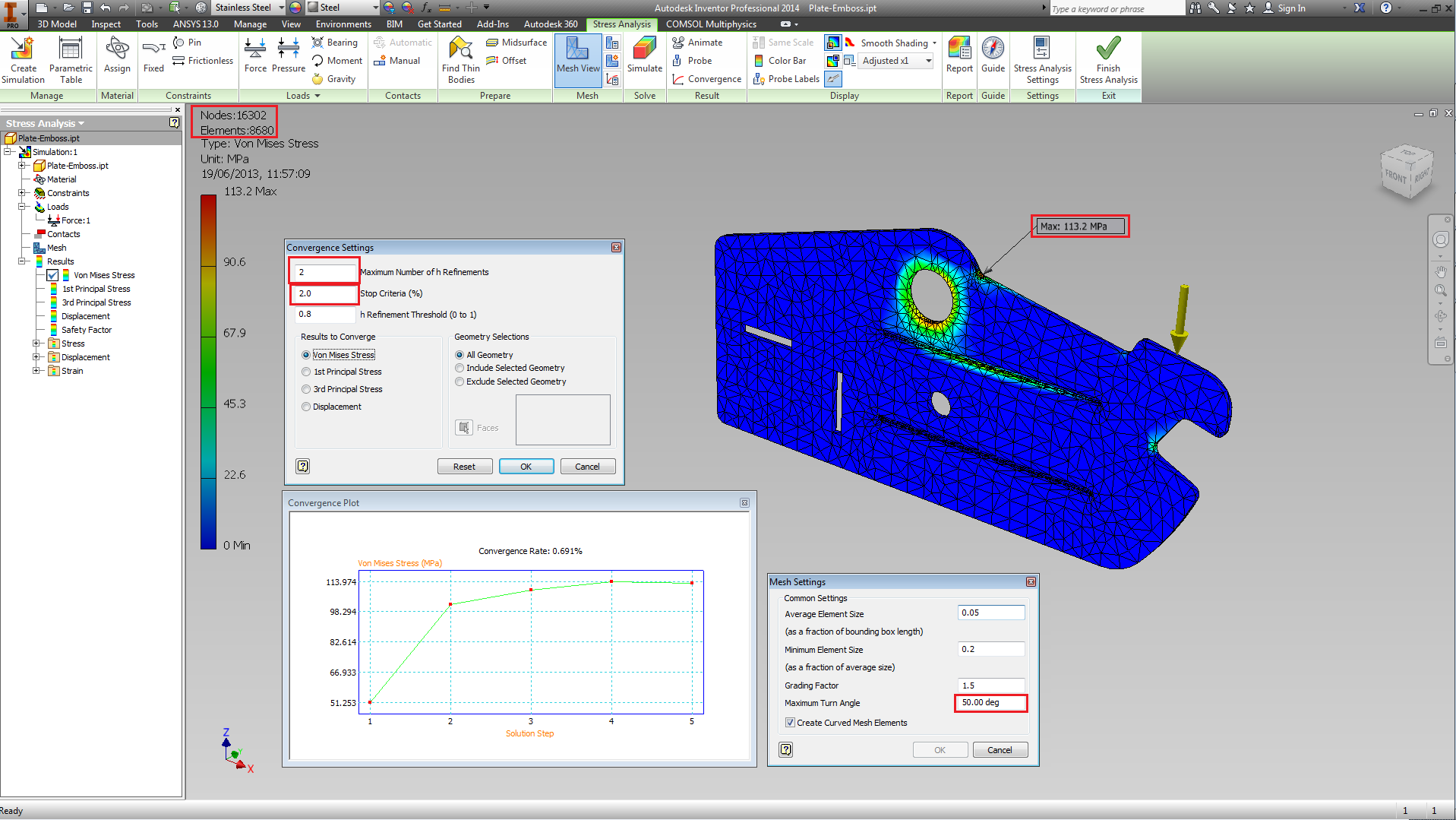8. ### Step 8:

Example 4

I rerun the analysis but I set the automatic refinement to 3 and next to 4.
What you need to check is...
Convergence Rate 0.084% (in a more complex model or assembly a percentage of below 10% is accurate enough).
The increment of the Elements, 13798
The stop Criteria is set to 0.1% -Too much confidence ;P
Max VM = 113.3 MPa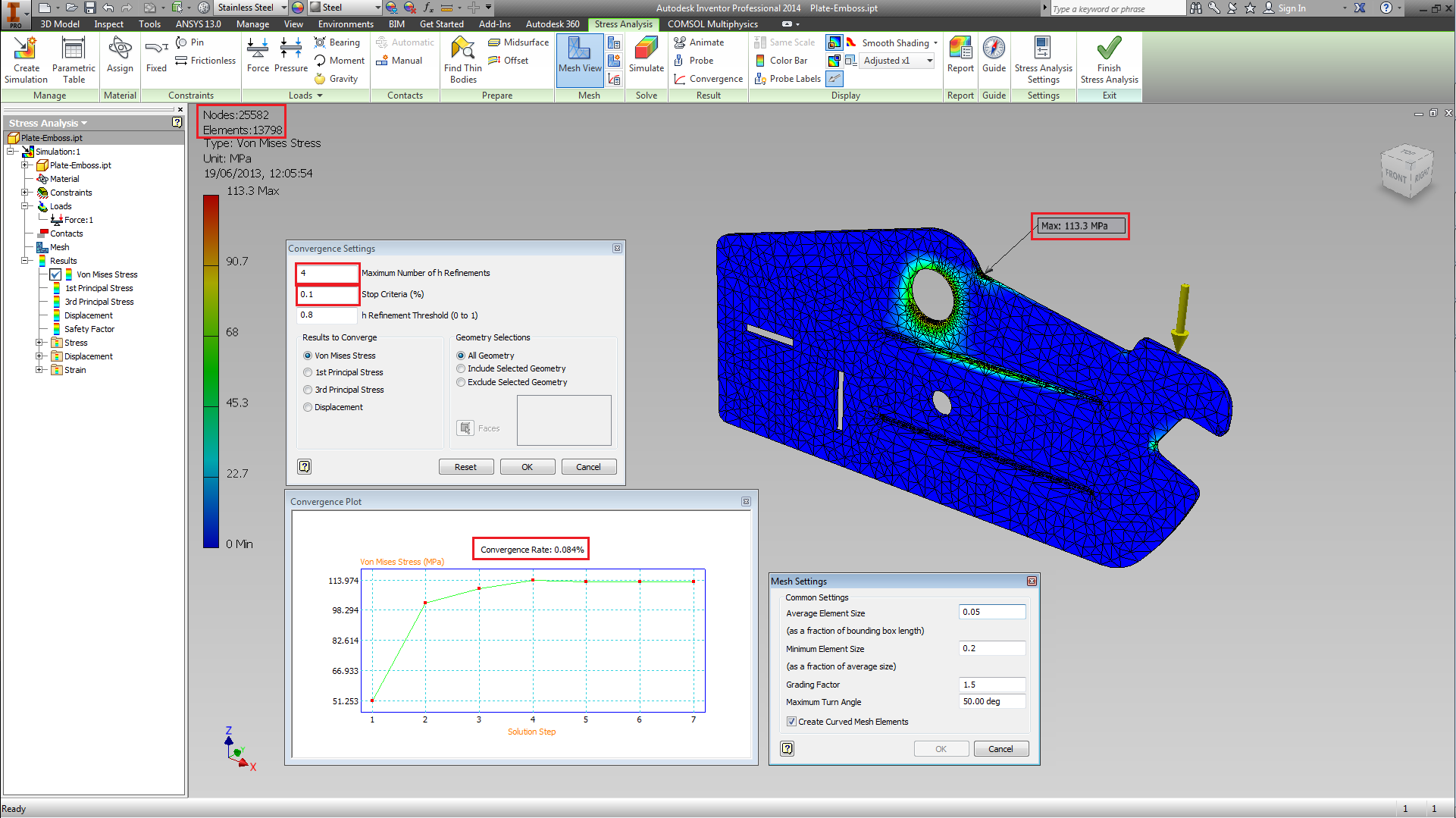9. ### Step 9:

Conclusion - Discussion

In conclusion the workflow of FEA it is simple. What it is not simple is to get valid results. In fact even these results of 113.3 MPa is just the best solution that you can get from the computation of the numerical model. The validation of results needs real experiments only then you will be 100% sure of what is happening & how far is your solution from the simulation.

Let me know if this tutorial enhanced your confidence against to FEA. At least now you know that the mesh is not a simple click and you can easily mistaken in your PREDICTIONS / RESULTS.

I hope you will enjoy it.

Regards

F.E.A. Rules ... Only when you know what to expect!!!

P.S. The solutions above represent Linear problem ...Yield Strength etc.